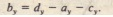# Sample Problem

Sample Problem

Gives an incomplete map of a road rally. From the starting point (at the origin). you must use available roads to go through the following displacements:

(I) a to checkpoint Able. magnitude 36 km, due east.
(2) b to checkpoint Baker. due north,
(3) c to checkpoint Charlie. magnitude 25 km, at the angle shown.
Your net displacement d from the starting point is 62:0 km.What is the magnitude b of b?

SOLUTION: The Key idea here is that the net displacement d is the vector sum of the three individual displacements. so we can writewhich gives usAlthough we know both magnitude and direction for a and C. we do not know both for d. so we cannot directly solve for b

However. we can write  of components along both the x axis and the y axis. Since points parallel to the y axis. choosing that axis may give us the b. We thus writeFollowing  inserting known data, and realizing that   b, we havewhich gives us,Inserting this into, we find.